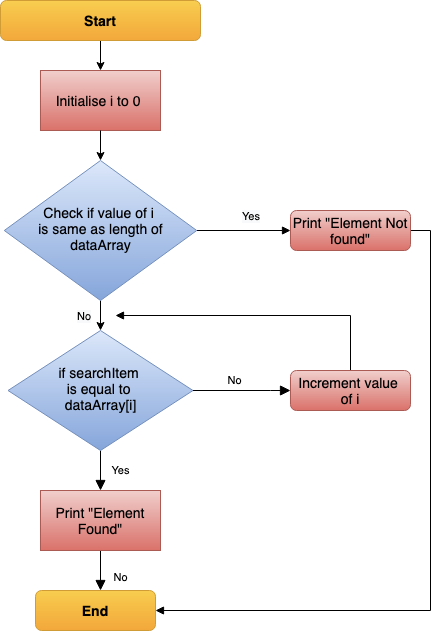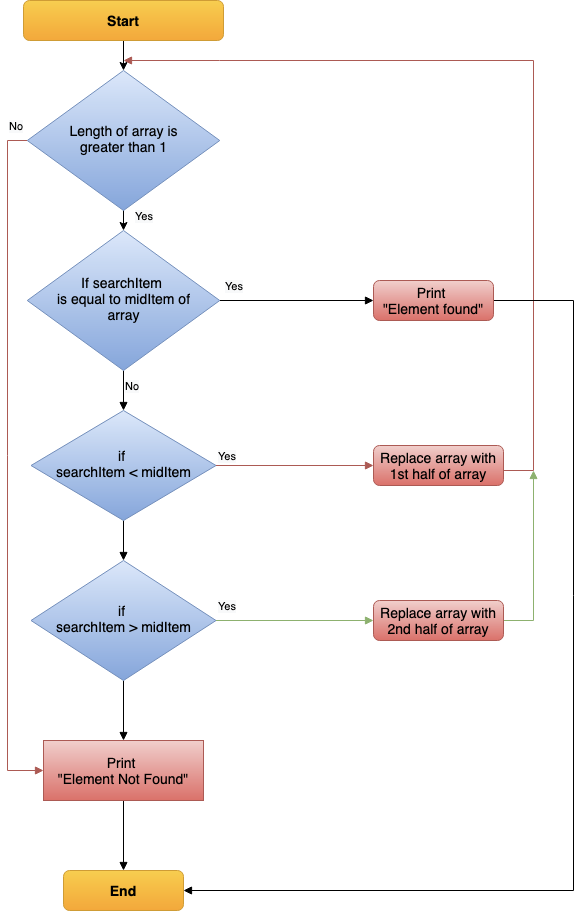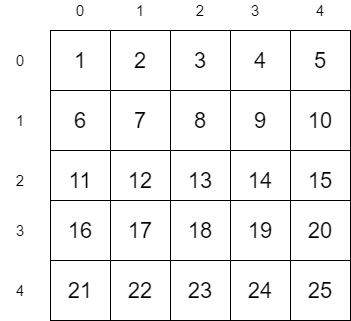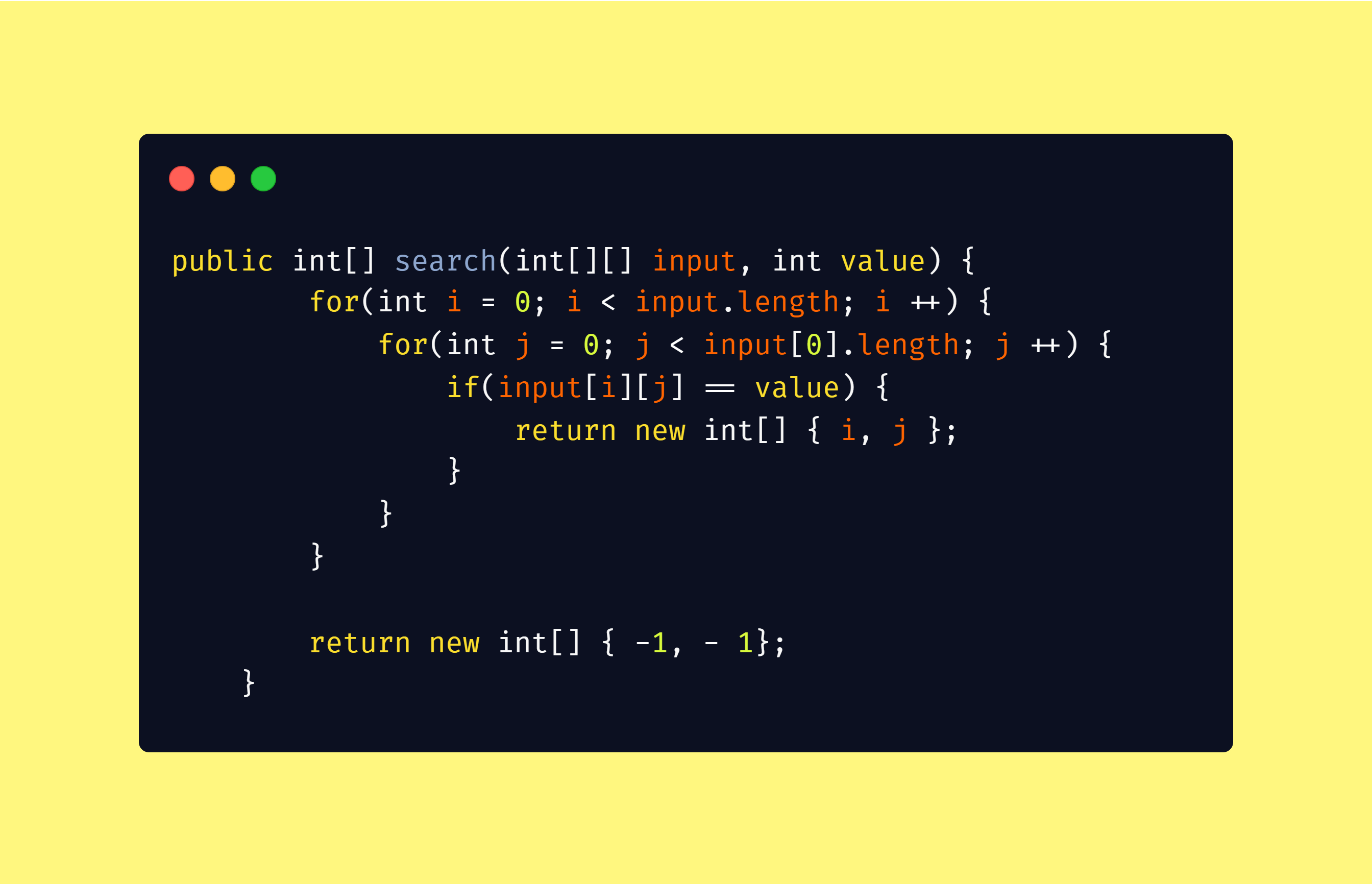1623681900

# How to Solve the LeetCode Algorithm Challenge: Search Insert Position

This task looks very simple, and at a first glance, it could be solved with the indexOf method. But this condition makes it trickier — “If the target isn’t found, return the index where it would be if it were inserted in order.”

So let’s break it down.

1. Compare each element of nums array to target
2. if matches return the index of matching element
3. if the element doesn’t match, check if the next element is less than the target if it’s not — return the next index position as a result.
4. Check if reached the end of the array and return position

#javascript

## Buddha Community1603767600

## Search Algorithms

Today, let us touch base on some fundamental concepts like search algorithms.

In simple terms, **searching **is a process of looking up a particular data record in the database or in the collection of items. A search typically answers as true or false whether the particular data in which we are referring is found or not and perform the next action accordingly.

Commonly used algorithms for search are:

• Linear search
• Binary search
• Interpolation search

Let us understand them in detail with some example

## Linear Search Algorithm

Linear Search Algorithm is the simplest and easiest form of the search algorithm. In this algorithm, a sequential search is made over all the items one by one to search for the targeted item. Each item is checked in sequence until the match is found. If the match is found, the searched item is returned otherwise the search continues till the end.

To make it easy to understand, let us see understand linear search using a flow diagramLinear Search — Data Flow

### Points to note:

• Does not need sorted array list
• Performs equality comparisons
• The time complexity is O(n)
• Time taken to search elements keeps increasing as the number of elements is increased.

## Binary Search Algorithm

In _Binary search algorithm, _begins with an interval covering the whole array and diving it in half. If the value of the search key is less than the item in the middle of the interval, narrow the interval to the lower half. Otherwise narrow it to the upper half. Repeatedly check until the value is found or the interval is empty.

To make it easy to understand, let us see understand binary search using flow diagram and example as below.Binary Search — Data Flow

### Points to note:

• The array needs to be sorted
• Performs ordering comparisons
• Time complexity to O(log n).
• Search is done to either half of the given list, thus cut down your search to half time

#sorting-algorithms #algorithms #data-structures #search-and-sort #searching-algorithm1596737880

# Intro

Binary Search is quite easy to understand conceptually. Basically, it splits the search space into two halves and only keep the half that probably has the search target and throw away the other half that would not possibly have the answer. In this manner, we reduce the search space to half the size at every step, until we find the target. Binary Search helps us reduce the search time from linear O(n) to logarithmic O(log n). But when it comes to implementation, it’s rather difficult to write a bug-free code in just a few minutes. Some of the most common problems include:

• When to exit the loop? Should we use `left < right` or `left <= right` as the while loop condition?
• How to initialize the boundary variables `left` and `right` ?
• How to update the boundary? How to choose the appropriate combination from `left = mid``left = mid + 1` and `right = mid``right = mid — 1` ?

A rather common misunderstanding of binary search is that people often think this technique could only be used in simple scenario like “Given a sorted array, find a specific value in it”. As a matter of fact, it can be applied to much more complicated situations.

After a lot of practice in LeetCode, I’ve made a powerful binary search template and solved many Hard problems by just slightly twisting this template. I’ll share the template with you guys in this post. **I don’t want to just show off the code and leave. Most importantly, I want to share the logical thinking: how to apply this general template to all sorts of problems. **Hopefully, after reading this post, people wouldn’t be pissed off any more when LeetCoding, “Holy sh*t! This problem could be solved with binary search! Why didn’t I think of that before!”

# Most Generalized Binary Search

Suppose we have a search space. It could be an array, a range, etc. Usually it’s sorted in ascend order. For most tasks, we can transform the requirement into the following generalized form:

Minimize k , s.t. condition(k) is True

The following code is the most generalized binary search template:

What’s really nice of this template is that, for most of the binary search problems, we only need to modify three parts after copy-pasting this template, and never need to worry about corner cases and bugs in code any more:

• Correctly initialize the boundary variables `left` and `right`. Only one rule: set up the boundary to include all possible elements;
• Decide return value. Is it `return left` or `return left — 1`? Remember this: after exiting the while loop, `**left**` is the minimal k​ satisfying the `**condition**` function;
• Design the `condition` function. This is the most difficult and most beautiful part. Needs lots of practice.

Below I will show you guys how to apply this powerful template to many LeetCode problems.

# Basic Application

## First Bad Version - LeetCode

You are a product manager and currently leading a team to develop a new product. Unfortunately, the latest version of…

leetcode.com

First, we initialize `left = 1` and `right = n` to include all possible values. Then we notice that we don’t even need to design the `condition` function. It’s already given by the `isBadVersion` API. Finding the first bad version is equivalent to finding the minimal k satisfying `isBadVersion(k) is True`. Our template can fit in very nicely:

## Sqrt(x) - LeetCode

Implement int sqrt(int x). Compute and return the square root of x, where x is guaranteed to be a non-negative integer…

leetcode.com

Quite an easy problem. We need to search for maximal k satisfying `k^2 <= x`, so we can easily come up with the solution:

There’s one thing I’d like to point out. Remember I say that we usually look for the minimal k value satisfying certain condition? But in this problem we are searching for maximal k value instead. Feeling confused? Don’t be. Actually, the maximal k satisfying `isBadVersion(k) is False` is just equal to the minimal k satisfying `isBadVersion(k) is True` minus one. This is why I mentioned earlier that we need to decide which value to return, `left` or `left — 1` .

#algorithms #leetcode #binary-search #algorithms1624874940

## Leetcodes Algorithm Series: Contains Duplicate

Hello, hello! As I continue my job search, I am practicing my algorithm questions on Leetcode. So, I thought I would blog about some Leetcode problems as I solve them.

As a Bootcamp graduate, I did not get much practice in algorithms, so it has been a very fun and sometimes frustrating trial and error in recognizing patterns, optimizing my code to be faster (looking at you Big-O), learning how to break down a problem, and sometimes implementing neat math tricks to solve these algorithm problems. I have been practicing them using JavaScript.

As I’m relatively new to algorithm problems, I’ve started out with their Easy Collection of Top Interview Questions. So in Contains Duplicate:

Given an integer array `nums`, return `true` if any value appears at least twice in the array, and return `false` if every element is distinct.

Examples:

``````Input: nums = [1,2,3,1]
Output: true

Input: nums = [1,2,3,4]
Output: false
Input: nums = [1,1,1,3,3,4,3,2,4,2]
Output: true
``````

Simply, if any numbers in the array appear more than once in the array, I need to return `true` . Now, I will definitely need to figure out a way to compare a number to the other numbers. Additionally, I will most likely need to loop through the array so I have access to each number to then compare it to others.

First, I thought that I could iterate through the array, and at each instance, I iterate through another loop of the remaining integers and compare them to see if any are equal (===). However, per my beginner understanding of Big-O, this would yield me an unfavorable time complexity of O(n²).

Then, I thought that I could create an object and count how many times a number in the array appears. By doing this, I could then check to see if any of the numbers had a count greater than 1. If so, then I could return `true` else I would return `false` .

#computer-science #leetcode #javascript #programming #algorithms #leetcodes algorithm1596737760

# Statement

We have to search for a value **x **in a sorted matrix M. If **exists, then return its coordinates **(i, j), else return (-1, -1).Let us consider the above matrix as an example. In this example, we are going to search for the value 12. Since 12 is present in the matrix, the algorithm should return its coordinates (2, 1)

# Simple Approach

A simple approach is to traverse all the values in the matrix and check if it is equal to 12.The worst case time complexity of the above algorithm will be

_O(n x m) = O(n²) __when _n = m

# Efficient Approach

The above algorithm behaves worse for large values of n and m. Let us look into the efficient algorithm now.

## Algorithm

``````1\. Start from Top Right position (0, m - 1) in the matrix M
2\. If the value is equal to x return (0, m - 1)
3\. Move one row down if the current value is less than x
4\. Move one column left if the current value is greater than x
``````

Let us apply this algorithm into our matrix M. We are going to search for the value 12 in M

#data-structures #search-sorted-matrix #2d-binary-search #algorithms #matrix-search #algorithms1597045745

## Searching Algorithms in Java

Searching Algorithms are designed to check for an element or retrieve an element from any data structure where it is stored. Based on the type of search operation, these algorithms are generally classified into two categories:

1. Sequential Search: In this, the list or array is traversed sequentially and every element is checked. For Example: Linear Search.
2. Interval Search: These algorithms are specifically designed for searching in sorted data-structures. These type of searching algorithms are much more efficient than Linear Search as they repeatedly target the center of the search structure and divide the search space in half. For Example: Binary Search.

Linear Search: The idea is to traverse the given array arr[] and find the index at which the element is present. Below are the steps:

• Let the element to be search be x.
• Start from the leftmost element of arr[] and one by one compare x with each element of arr[].
• If x matches with an element then return that index.
• If x doesn’t match with any of elements then return -1.

#java #searching #algorithms-searching #binary search